## research papersJOURNAL OFSYNCHROTRONRADIATION
ISSN: 1600-5775
Volume 25| Part 6| November 2018| Pages 1650-1657

## Subpixel resolution in CdTe Timepix3 pixel detectors

aDepartment of Physics, NEXMAP Section, Technical University of Denmark, Fysikvej, Lyngby, Copenhagen 2800, Denmark, bDepartment of Physics, University of Copenhagen, Nørregade 10, Copenhagen 1165, Denmark, and cAdvacam s.r.o., U Pergamenky 1145/12, 17000 Prague 7, Czech Republic
*Correspondence e-mail: mohkha@fysik.dtu.dk

(Received 28 February 2018; accepted 29 September 2018; online 26 October 2018)

Timepix3 (256 × 256 pixels with a pitch of 55 µm) is a hybrid-pixel-detector readout chip that implements a data-driven architecture and is capable of simultaneous time-of-arrival (ToA) and energy (ToT: time-over-threshold) measurements. The ToA information allows the unambiguous identification of pixel clusters belonging to the same X-ray interaction, which allows for full one-by-one detection of photons. The weighted mean of the pixel clusters can be used to measure the subpixel position of an X-ray interaction. An experiment was performed at the European Synchrotron Radiation Facility in Grenoble, France, using a 5 µm × 5 µm pencil beam to scan a CdTe-ADVAPIX-Timepix3 pixel (55 µm × 55 µm) at 8 × 8 matrix positions with a step size of 5 µm. The head-on scan was carried out at four monochromatic energies: 24, 35, 70 and 120 keV. The subpixel position of every single photon in the beam was constructed using the weighted average of the charge spread of single interactions. Then the subpixel position of the total beam was found by calculating the mean position of all photons. This was carried out for all points in the 8 × 8 matrix of beam positions within a single pixel. The optimum conditions for the subpixel measurements are presented with regards to the cluster sizes and beam subpixel position, and the improvement of this technique is evaluated (using the charge sharing of each individual photon to achieve subpixel resolution) versus alternative techniques which compare the intensity ratio between pixels. The best result is achieved at 120 keV, where a beam step of 4.4 µm ± 0.86 µm was measured.

### 1. Introduction

Since 2006 photon-counting (PC) detectors have surpassed scintillating detectors for many applications of X-ray diffraction. The low-noise floor and large dynamic range has led to this transformation. Recently we have seen the functionality of solid-state detectors increase to include spectral information. Again, the diffraction measurements were well suited to see the first implementations (O'Flynn et al., 2013). First of all, the increased pixel sizes, typically required in this type of detector, can be mitigated by increasing the sample-to-detector distance. Furthermore, the low intensity of the diffracted signal matches the limitations in flux handling capabilities of the PC detectors, not to mention that the spectral resolution allowed the diffraction experiments to be made with polychromatic laboratory sources. Subsequently, as this next generation of PC detectors could handle higher and higher flux densities (Rinkel et al., 2011), they have moved to the field of tomography (Kheirabadi et al., 2017). For tomography, the energy resolution has been used to improve the handling of beam hardening and to improve material contrast (Olsen et al., 2017) and for revealing quantitative information about the elemental composition of the absorber in K-edge imaging (Roessl & Proksa, 2007). While superior in these areas, spatial resolution remains an area where the PC detectors cannot compete with other technologies; so far the smallest pixel sizes have been tens of micrometres. Another challenge of these detectors is found when these detectors are used for hard X-ray beams (>20 keV), where the typical sensor material is GaAs, CdTe or CZT for the sake of efficiency and ability to operate at room temperature. The spectroscopic performance of these detectors is then limited by the effects of charge sharing between neighboring pixels and the high ratio of intrinsic characteristic X-rays from the sensor (Taguchi & Iwanczyk, 2013; Dreier et al., 2018; Trueb et al., 2017; Khalil et al., 2018).

Hybrid pixel detectors from the Medipix collaborations (http://medipix.web.cern.ch/) were developed to replace striped detectors in the inner tracking layers of the vertex detectors at the Large Hadron Collider. A history of the early developments of pixel detectors can be found in Heijne (2001). Two distinctive streams of development can be identified: the Medipix family of ASICs which integrate data from multiple hits on a pixel and provide the images in the form of frames, and the Timepix family of ASICs which aim to send as much information about individual interactions as possible. Currently, there are two Timepix ASICs, namely Timepix (Llopart et al., 2007) and Timepix3 (Poikela et al., 2014). Table 1provides a comparison between the main specifications of Timepix3 and Timepix.

 Table 1A comparison between the main specifications of Timepix3 and Timepix
 The major differences are found in the measurement modes and the data readout modes. Operating Timepix3 in `energy (ToT) + time-of-arrival (ToA)' measurement mode allows for the unambiguous identification of clusters belonging to single X-ray interactions up to 40 Mcounts s−1.
Timepix3 Timepix
Pixel matrix 256 × 256 256 × 256

Pixel pitch 55 µm 55 µm

Measurement modes (i) ToT + ToA (640 MHz) (i) Integrated ToT
(ii) ToA (640 MHz) (ii) ToA (up to 100 MHz)
(iii) Events + integrated ToT (iii) Events

Data readout modes (i) Frame based (up to 1700 frames s−1) (i) Frame based (up to 850 frames s−1)
(ii) Data-driven (up to 40 Mcounts s−1)

With the Timepix chip, it is possible to measure the total charge deposited in a cluster of pixels. Thus, it is possible to correct for the charge-sharing effect in pixel detectors (Jakubek, 2009) and bin the detected photon according to the total charge deposited. However, when the Timepix chip is operated in energy mode (ToT: time-over-threshold), the primary limitation comes from the need to avoid overlapping clusters from different interactions. In practice, this reduces the number of clusters per frame and hence the frame length. The maximum frame rate in Timepix is 850 s−1, which limits the flux to 850 photons s−1. In addition, the Timepix chip is insensitive during the frame readout and this imposes restrictions on the applications (Ballabriga et al., 2018). Timepix3 overcomes these limitations by using a data-driven architecture with data treatment of up to 40 Mcounts s−1, where each hit pixel immediately initiates the sending of its data off-chip. The data contain information on the ToT and time-of-arrival ToA. The ToA information allows the unambiguous identification of pixel clusters belonging to a single X-ray interaction. Once identified, the ToT of the pixel cluster is summed and the photon is assigned to the brightest pixel in the cluster. The spatial resolution of Timepix3 can be further improved by taking advantage of the charge-sharing effect. Instead of assigning the photon to the brightest pixel, the weighted mean of the energy distribution in the pixel cluster is used to localize the subpixel position of the X-ray interaction. A higher spatial resolution will further increase the relevance of semiconductor hybrid pixel detectors for applications in attenuation tomography and diffraction tomography (Cersoy et al., 2015).

In this work, we present a study on the subpixel resolution response of a 1 mm-thick CdTe-based Advapix-Timepix3 detector. We scanned an ADVAPIX-Timepix3 pixel (55 µm × 55 µm) using a pencil beam (5 µm × 5 µm) in steps of 5 µm at four monochromatic energies (24, 35, 70 and 120 keV). Given the small size of the pixels compared with the thickness of the CdTe sensor, X-rays in the considered energy range are read by clusters of up to six pixels. We measure the beam subpixel position using the cluster information for single-photon interactions. We present the optimum conditions for the subpixel measurements with regard to the cluster sizes and the subpixel position of the beam. Finally, we evaluate the functionality of this approach (using the charge sharing of each individual photon to achieve subpixel resolution) versus alternative techniques which compare the intensity ratio between pixels, where each photon is only registered as belonging to a single pixel.

### 2. Experiment

The experiment was conducted at the high-resolution diffractometer beamline ID11 at the European Synchrotron Research Facility (ESRF) in Grenoble, France. We used a 5 µm × 5 µm monochromatic pencil beam at four energies, 24, 35, 70 and 120 keV, to scan an ADVAPIX-Timepix3 pixel. ADVAPIX-Timepix3 is a new hybrid pixel detector produced by ADVACAM s.r.o., Prague, Czech Republic, and the one used for this experiment is one of the first manufactured models. ADVAPIX is formed of a 1 mm-thick CdTe sensor that is readout by a Timepix3 ASIC. The detector was operated in ToT + ToA measurement mode where the data are read out in data-driven mode.

The X-ray intensity on the detector was tens to hundreds of detected photons s−1 which corresponds to fluxes of 0.4 to 4 × 106 photons s−1 mm−2. The energy resolution of the monochromatic X-ray beam was measured using an Amptek XR100T CdTe pin detector (Amptek Inc., Bedford, USA). The full width at half-maximum (FWHM) was determined to be below the 1.4 keV resolution limit of the Amptek detector (Dreier et al., 2018). An X-ray beam energy FWHM of 1.4 keV is well below the energy resolution of a CdTe Timepix3 Advapix detector.

The detector was placed at a position where the beam illuminated the edge of the pixel (128, 206) (position 1 in Fig. 1) at 90° with respect to the detection surface. The target pixel was selected randomly. The initial position of the beam was intended to be at the intersection of four neighboring pixels. This initial position was estimated by moving the detector in small increments until the four pixels surrounding the beam read signals of the same order of magnitude. The detector was stepped in increments of 5 µm to scan pixel (128, 206) in an 8 × 8 matrix and produce a data set representing the response of the whole pixel as shown in Fig. 1. The scan did not cover the full pixel because we assumed that the response was symmetric. At each position, 10 s of data were acquired. The data contain information on the position, energy and time stamp of all hit pixels during the 10 s.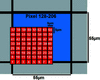Figure 1 Map of the expected beam position on the pixel (128, 206). A 5 µm × 5 µm beam illuminates the studied pixel head on. Position 1 represents the initial beam position, which is meant to be at the intersection of four pixels. The detector is then moved by increments of 5 µm as represented by the position numbers on the figure. These position numbers are referred to as the subpixel beam-position index.

We will use the subpixel analysis algorithm, described in §3.2, in order to measure the 8 × 8 subpixel positions shown in Fig. 1. We will compare the results at different energies to find the optimal conditions for the subpixel measurements.

### 3. Analysis

#### 3.1. Charge sharing and formation of pixel clusters

The dominant interactions for X-rays in the region 20–120 keV are photoelectric absorption and Compton scattering. When an interaction occurs, the X-ray transfers its energy (or a portion of it in the case of Compton scattering) to an orbital electron belonging to an atom of the detector sensor. The electron is promptly ejected from the atom carrying the deposited energy and loses its kinetic energy by ionizing neighboring atoms. This results in the creation of N mobile electron/hole pairs proportional to the deposited energy Ei,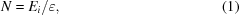whereis the average ionization energy required to produce one electron/hole pair.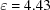eV for CdTe (Knoll, 2000).

An applied bias voltage generates an electric field within the detector volume, causing the induced charge clouds to drift towards the collecting electrodes/pixels. Charge induction on the electrodes/pixels is caused by this drift and is proportional to the energy deposition of the X-ray (Shockley, 1938; Ramo, 1939). The drift of the charge clouds is accompanied by diffusion and Coulomb's repulsion that spread the charge out and may lead to charge sharing. Charge sharing occurs when the photon energy is read out by multiple electrodes/pixels. There are multiple factors that affect charge sharing. The energy transferred from the photon to an electron influences the initial volume of the charge cloud and the number of electron/hole pairs created. The position of the interaction determines the drift length and thus the cloud volume. The applied biasing voltage as well as the carrier mobilities affect the charge-collection speed and the charge-recombination rate.

#### 3.2. Subpixel measurement algorithm

The response of the detector caused by 120 keV photon interactions is shown in Fig. 2for pixel clusters sizes of one to six. We did not consider larger cluster sizes in our analysis because of their low count statistics. The subpixel resolution is then retrieved using the following procedure.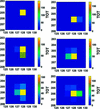Figure 2 Pixel clusters caused by a 120 keV photon interaction. Interacting X-rays are detected by one or more pixels because of the charge-sharing effect in pixel detectors (Jakubek, 2009). The scale represents time-over-threshold data (arbitrary energy units).

(i) Segmenting by time-of-arrival: by utilizing Timepix3's time-of-arrival stamping it is possible to extract the pixels that are engaged simultaneously. The charge collection time is of the order of tens of nanoseconds. The time-of-arrival resolution is 1.56 ns. We use a time window of 20 ns because the pixels belonging to the same cluster do not necessarily read data simultaneously. As the charge cloud drifts towards the electrodes, it increases in volume. Hence, some pixels in the cluster can read data later than others. Typically the brightest pixels in the cluster are those that start reading first. The least bright pixels are those that read data last.

(ii) Clustering hit pixels: neighboring pixels that are hit within the time window of 20 ns, are clustered. The considered clusters are those with a size of 1, 2, 3, 4, 5 or 6 pixels.

(iii) Identifying the central pixel for every photon: the central pixel is the brightest pixel in the cluster. This is done for every single photon.

(iv) Calculating each photon position: considering the photons that engage with more than one pixel, it is possible to find a subpixel position for every photon by finding the weighted means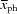and/or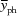of the photons' positions in the first and second dimensions,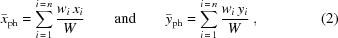where n is the magnitude of the pixel cluster in the event, wi is the ToT signal on the pixel, W is the total ToT of the event and (xi, yi) represents the pixel position in the 256 × 256 pixel matrix. We only consider the first and second neighbor pixels around the central pixel, which is sufficient for the considered pixel-cluster sizes (up to 6-pixel clusters).

(v) Calculating the beam subpixel position: after calculating the weighted-mean position for every photon in a beam, the beam position is found by calculating the average of the weighted means.

### 4. Results and discussion

The discussion presented in this section covers three points: (i) the dependence of the pixel-cluster distribution on the subpixel beam position and energy; (ii) the dependence of the beam-step measurement on the subpixel beam position and energy; and (iii) the dependence of the beam-step measurement on the pixel-clusters size. Finally, we present an evaluation of the precision in the subpixel measurement obtained.

#### 4.1. Pixel-cluster distribution versus beam position and energy

In order to evaluate the dependence of the pixel-cluster distribution on the subpixel beam position and energy, we plotted the pixel-cluster distribution at selected beam index positions in Fig. 3. These positions represent points of interest such as the center of a pixel, the edge between two pixels (referred to as 2-pixel-edge hereafter) and the cross-point between four pixels (referred to as 4-pixel-edge hereafter). A strong dependence on the beam position and energy is observed. There is an increase in large pixel-cluster sizes when the beam illuminates the edge between two or four pixels. In contrast, the pixel-cluster distributions shift towards smaller pixel-cluster sizes when the beam illuminates the center of the pixel. This behavior is attributed to charge clouds drifting in the vicinity of multiple pixels. The number of involved pixels increases or decreases depending on the beam position. In addition, the figure shows a progressive increase in the number of pixel-cluster sizes as the energy is increased. This is attributed to the increased number of electron/hole pairs created which results in larger charge-cloud volumes (Jakubek, 2009).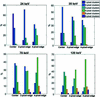Figure 3 Pixel-cluster distribution at different positions and different energies. Center represents the case where the beam is at the center of the pixel (example: position 55 on Fig. 1), 2-pixel-edge represents the case where the beam is close to the edge between two pixels (example: position 8 on Fig. 1) and 4-pixel-edge represents the case where the beam is close to the edge between four pixels (example: position 1 on Fig. 1).

#### 4.2. Measured beam positions versus expected beam positions and energy

In this section, we study the measured beam positions dependence on the expected beam positions and energy. The expected beam positions are the positions of the beam after stepping the detector translation motor. The expected step, which is the difference between two consecutive beam positions, is 5 µm. The measured beam positions were calculated using the approach in §3.2and are plotted in Fig. 4. For this measurement, we used all pixel clusters (1 to 6). The starting position of the beam is not the same for different energies, because there was a need to perform a realignment of the beam with respect to the pixel whenever the energy was changed.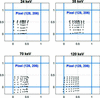Figure 4 Measured subpixel beam positions on the pixel (128, 206) using all pixel clusters at different energies. The expected beam position is mapped in Fig. 1. The starting position is not the same for different energies because there was a need to realign the beam after every change in the beam energy.

Because of the lack of an absolute reference for beam position, the accuracy is inferred from the relative distance between beam positions. To evaluate the measured beam positions (Fig. 4), we plotted the measured steps along the x and y directions in Fig. 5. The measured steps can be inferred from Fig. 1, where the first measured step along the x direction and its error bar are the mean and standard deviation of the following steps: (1 to 2), (9 to 10), (17 to 18), (25 to 26), (33 to 34), (41 to 42), (49 to 50) and (57 to 58). The second measured step along the x direction and its error bar are the mean and standard deviation of the following steps: (2 to 3), (10 to 11), (18 to 19), (26 to 27), (34 to 35), (42 to 43), (50 to 51) and (58 to 59). Similarly, the rest of the measured steps and their error bars can be inferred. Since the beam was moved in 64 positions covering an 8 × 8 matrix, there are seven steps along the x and y directions, respectively. These measured steps are plotted versus the expected beam position in Fig. 5. The expected step is a constant of 5 µm for all the steps. However, the measured steps are not constant and they show a dependence on the expected beam position. The measured steps are largest close to the pixel border and become progressively smaller as the expected beam position moves towards the pixel center. Close to the pixel center, we observe that the measured steps reach their minimum values. In some cases, where the beam crosses the pixel center towards the other edge of the pixel, we observe that the measured step starts to increase again (for example, y direction at 24 keV and 35 keV in Fig. 5). This behavior is observed at all the considered energies. However, the deviation of the measured step from the expected step of 5 µm decreases as the energy is increased. To study the energy dependence, we considered the maximum and minimum values of the measured steps and observed their change as a function of energy. At 24 keV, the corresponding values are 1.5 and 8 µm. At 35 keV, there is a slight improvement and the corresponding values are 1.5 and 7 µm. At 70 keV, more improvement is observed and the corresponding values are 1.8 and 6.8 µm. The relatively large error bars at 70 keV are due to the considerably lower statistics at 70 keV where the photon flux dropped to tens of photons s−1. The reason for this is the tight collimation of the beam which resulted in a reduction in the flux. We only observed this after the experiment. At 120 keV, we observe the best correlation between the measured step and the expected step size. The minimum and maximum values for the measured step are 3 and 5.9 µm, respectively. This progressive improvement is attributed to the increase in large pixel clusters as the energy is increased (refer to Fig. 3), which is discussed in the following section. This increase in large pixel clusters allows for better photon subpixel measurement. The dependence of the measured steps on the cluster sizes is studied in §4.3.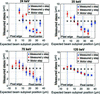Figure 5 The measured steps can be inferred from Fig. 1, where the first measured step along the x direction and its error bar are the mean and standard deviation of the following steps: (1 to 2), (9 to 10), (17 to 18), (25 to 26), (33 to 34), (41 to 42), (49 to 50) and (57 to 58). Similarly, the second measured step and its error bar are the mean and standard deviation of the following steps: (2 to 3), (10 to 11), (18 to 19), (26 to 27), (34 to 35), (42 to 43), (50 to 51) and (58 to 59). The x-axis represents the expected position of the beam inside the pixel where position 0 µm is the pixel edge (pixel start) and 27.5 µm is the pixel center. The initial position of the beam is not always at the start of the pixel as concluded from Fig. 4, hence the positions were adjusted using the first beam position as measured in Fig. 4.

The best result is achieved at 120 keV, where we measured a beam step of 4.4 µm ± 0.86 µm. In addition, if the pixel scan continued from the pixel (128, 206) center to the other pixel (128, 206) edge, we expect that this result will improve further. We expect that the measured step is a minimum close to the center and that it starts to increase progressively until it reaches its maximum values again at the second pixel edge.

#### 4.3. Measured beam positions versus cluster sizes

The charge-sharing effect, which leads to cluster formation, has been described by Jakubek (2009). The charge is measured by every pixel with the ToT method. The charge collected by the pixels is compared against a threshold. If the charge is lower than the threshold then it is not registered, resulting in charge loss and distortions in the subpixel measurements. This effect is more significant at small clusters where the charge loss is relatively high compared with the total deposited charge.

For the evaluation of the correlation between the measured step size and the pixel-cluster size only data acquired with a beam energy of 120 keV were utilized, since they yield sufficient statistics for all analysed cluster sizes from 2 to 6 pixels. In Fig. 6and Fig. 7we show the obtained beam position and measured steps using different single pixel-cluster sizes.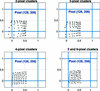Figure 6 Measured beam subpixel position using different clusters at 120 keV. The expected beam position is mapped in Fig. 1. The starting position is not the same for different energies because there was a need to realign the beam after every change in the beam energy.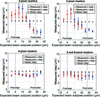Figure 7 Dependence of the measured step on the expected beam position using different pixel clusters at 120 keV. The measured steps can be inferred from Fig. 1, where the first measured step along the x direction and its error bar are the mean and standard deviation of the following steps: (1 to 2), (9 to 10), (17 to 18), (25 to 26), (33 to 34), (41 to 42), (49 to 50) and (57 to 58). Similarly, the second measured step and its error bar are the mean and standard deviation of the following steps: (2 to 3), (10 to 11), (18 to 19), (26 to 27), (34 to 35), (42 to 43), (50 to 51) and (58 to 59). The x-axis represents the expected position of the beam inside the pixel where position 0 µm is the pixel edge (pixel start) and 27.5 µm is the pixel center.

At small cluster sizes (2- and 3-pixel clusters) the measured step has a similar behavior to that observed in Fig. 5, where the measured step has a maximum size close to the pixel border and a minimum size close to the pixel center. The minimum and maximum values of the measured steps are 2 and 8.5, respectively. In contrast, large cluster sizes (4-, 5- and 6-pixel clusters) show a more uniform measured step of about 4 µm. The minimum and maximum values of the measured steps are 3.8 and 4.8, respectively. This is in line with the behavior of energy dependence observed in Fig. 4. At low energies (24 keV and 35 keV), the pixel-cluster distribution is dominated by 2 and 3-pixel clusters sizes. At high energies (120 keV), the majority of the pixel clusters are of sizes 3 and 4 (see Fig. 3). This results in a weaker dependence on the pixel-edge distance and more uniformity in the measured steps. We observe a high standard deviation in the 5- and 6-pixel cluster plot compared with the 4-pixel cluster plot. This is possibly due to the effect of k-shell fluorescence of both Cd and Te used as the detector sensor. When a k-shell fluorescence photon is emitted, it has a high probability to be absorbed within two pixels from the initial interaction. This results in a distortion of the cluster, which affects the subpixel measurement.

According to our results, the best step measurement is achieved at 120 keV by only using 4-pixel clusters. However, even under these optimal conditions the measured step is only 4 µm, and thus lower than the motor encode estimate of 5 µm. A scan of an entire pixel or even a number of adjacent pixels could provide useful information to investigate the discrepancy further; however, the acquisition of the required data was not feasible in the limited time allocated for the experiment at the beamline.

#### 4.4. Evaluation versus other methods

In the following, we evaluate the precision of the measurement. To distinguish between the precision and the accuracy of a measurement we denote the standard deviation of repeated position measurements as a measure of precision, while the deviation from the position determined from motor positions is the accuracy (Mortensen et al., 2016). The data are treated as a sampling distribution, where the population is the measured subpixel position of every single photon in the beam. First, we will compute the standard deviation of the population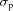(accuracy of single-photon measurements contributing to the mean of the beam). Then, we will compute the standard deviation of the sampling distribution of the mean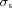. The termshows how many photons are needed to measure the center of the beam within a defined error.

The variance of the single photons contributing to the position of the beam depends on both position and energy. We calculated the standard deviation of the population as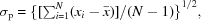where N is the number of photons in the beam for a position and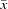is the mean position of all photons. The standard deviation of the measurement is shown for 120 keV at all 64 beam positions in Fig. 8. For our data set, the standard deviation or precision in the y position is approximately 0.35 pixels (19.25 µm) regardless of beam position in the corner, centrally on an edge or in the center of a pixel as seen in Fig. 8. The standard deviation in x position is approximately 0.28 pixels (15.4 µm). It is not clear why we achieve 25% better precision in one direction over the other. This possibly requires testing a line of pixels and testing pixels in different areas of the detector to judge whether this effect is caused by the source or the detector. The precision of the measured position when measuring on just a single photon is thus comparable with the pixel size for all combinations of positions and photon energy, which limits the effectiveness of single-photon measurements. In this context, the contribution to the uncertainty from the 5 µm width of the beam size is negligible.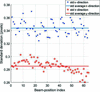Figure 8 Standard deviation of the measured beam position at 120 keV. The beam-position index is mapped in Fig. 1.

In the previous paragraph, we computed the standard deviation of the population (accuracy of single-photon measurements contributing to the mean of the beam). Now we will compute the standard deviation of the sampling distribution of the mean to obtain a measure on the subpixel resolution of a beam with a larger number of measured photons, and compare the method with other approaches to obtain subpixel resolution. This approach shows how many photons are needed to measure the center of the beam within a defined error. According to the variance sum law,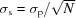, whereis the standard deviation of the measured population of photons andis the standard deviation of the sampling distribution of the mean of a population of N photons;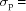19.25 µm as computed in the previous paragraph. Then, for a sample size of 1000 photons, the standard deviation of the measured beam position will thus be decreased to= 0.6 µm for the sample population. The deviation of this subpixel method can be compared with an alternative technique of comparing the intensity ratio between pixels. For this method, each photon is only registered as belonging to a single pixel and the algorithm calculates the ratio of intensity between two pixels to infer the midpoint of the beam (Krejci et al., 2011). For the beam geometry used here, the method would work very well for beam positions close to the edge of the pixels. However, the beam is so narrow that when it illuminates the center of a pixel only a very small percentage of photons hits the neighbor pixel. In that case, the shot noise fluctuation would give the method a very high variance. Contrary to the method we are presenting, the precision is thus not the same for all beam positions. As an example, if we calculate the variance for a beam position halfway between the pixel center and the pixel edge such as position 52 in Fig. 1, the ratio of photon counts between the neighboring pixels is 0.994. The precision would be a function of the variation of the number of photons collected in the neighbor pixel with the lowest count. Monte Carlo simulation shows that the total number of photons in the beam would have to be larger than 10000 for this subpixel technique to reach the same standard deviation as the method we present.

### 5. Conclusion and outlook

The functionality of the Timepix3 for analysing the charge collection of each individual photon shows a consistently better precision of beam positioning compared with methods available with photon-counting detectors. These results are also achievable with a lower number of photons (1/10) making it possible for use with fast acquisitions and where weak high-resolution sources are used. Because of the lack of an absolute reference for beam position the accuracy is inferred from the relative distance between beam positions. The best result was obtained by using 120 keV photons, where we measured a beam step of 4.4 µm ± 0.86 µm. The measured beam step showed a dependence on the position on the pixel. The measured step is maximum near the pixel edge and minimum near the pixel center. We did not scan the full pixel from one side to the other.

We are currently exploring the use of laboratory X-ray polychromatic sources where we can utilize Timepix3 to its full potential (energy dispersion and fine spatial resolution). A local setup would give us the time needed for longer scans of both full pixels and for exploring the repeatability of multiple pixels across the sensor. Further studies also include investigating the influence of the bias voltage on subpixel resolution as well as correcting for intrinsic X-ray fluorescence emission from the CdTe sensor, which is suspected to distort large pixel clusters.

### Acknowledgements

The authors would like to acknowledge the MEDIPIX collaborators who are responsible for the development of the Timepix3 ASIC used in this work. Furthermore, we thank Jonathan Wright from ID-11 for his assistance during the beam time.

### Funding information

The authors would like to thank Exruptive for financing the ESRF beam time through the initiative LINX (Linking Industry with Neutrons and X-rays). The authors acknowledge Innovation Fund Denmark and the EUROSTARS programs for financing their research work.

### ReferencesJOURNAL OFSYNCHROTRONRADIATION